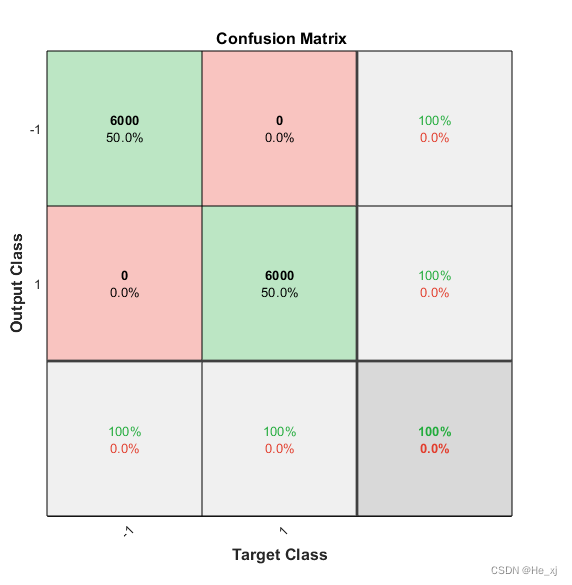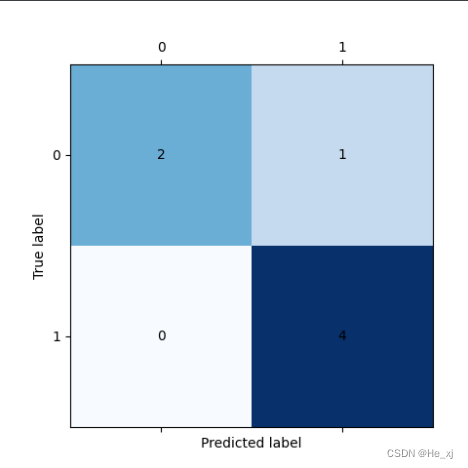## 程序员最近都爱上了这个网站  程序员们快来瞅瞅吧！  it98k网:it98k.com

出租广告位,需要合作请联系站长

+关注

## 绘制混淆矩阵(MatLab/Python)

其实混淆矩阵就是用来判断我们的算法的分类准确度的一个可视化矩阵

## 1.使用matlab绘制混淆矩阵

可以使用函数 plotconfusion 来绘制混淆矩阵

``plotconfusion(y_true, y_predict)``

``predict_label = predict(SVMModel, data);y_true = categorical(label);y_predict = categorical(predict_label);plotconfusion(y_true, y_predict)``## 2.使用Python绘制混淆矩阵

``sklearn.metrics.confusion_matrix(y_true, y_pred, *, labels=None, sample_weight=None, normalize=None)``
``from sklearn.metrics import confusion_matriximport matplotlib.pyplot as plt y_pred = [] # ['2','2','3','1','4'] # 类似的格式y_true = [] # ['0','1','2','3','4'] # 类似的格式# 对上面进行赋值C = confusion_matrix(y_true, y_pred) plt.matshow(C, cmap=plt.cm.Blues) # 根据最下面的图按自己需求更改颜色for i in range(len(C)):    for j in range(len(C)):        plt.annotate(C[j, i], xy=(i, j), horizontalalignment='center', verticalalignment='center') plt.ylabel('True label')plt.xlabel('Predicted label')plt.show()``8 0

pdf(new) 更多>